# Algebra II : Algebra II

## Example Questions

### Example Question #10 : Binomial Theorem

Which is equivalent to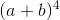?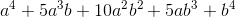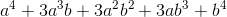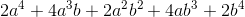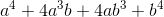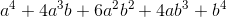Explanation:

To answer this question, you could either use the binomial theorem or multiply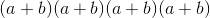. Both are detailed below.

Note: If you need help understanding Sigma Notation, please visit: https://www.varsitytutors.com/hotmath/hotmath_help/topics/sigma-notation-of-a-series; additionally, the notationis often read out loud as “n choose k” and is another way to writeFor more information on combinations, visit https://www.varsitytutors.com/hotmath/hotmath_help/topics/combinations

The Binomial Theorem is:In our case, n=4. Plugging this in, we get:Alternatively, we can solve this problem by multiplying to get the following: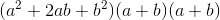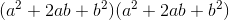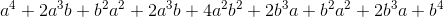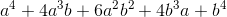### Example Question #1 : Representing Data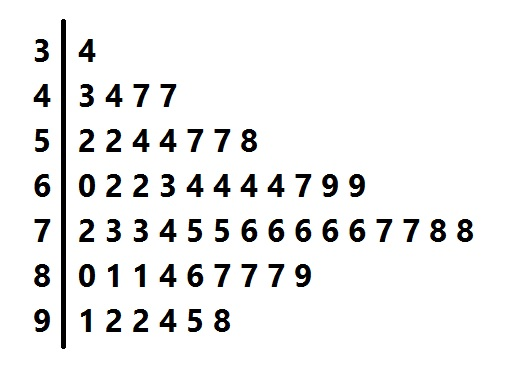Above is a stem-and-leaf representation of the scores on a test administered to a group of students. What was the mode of the scores?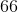The set of scores has more than one mode.Explanation:

The mode of the data set is the score that occurs the most frequently.

Each "stem" in the left column represents the tens digits of the scores; each of the numbers in its row, or "leaf" represents the units digits. The entry that occurs the most frequently is represented by the "6" in the "7" row, so 76 is the one and only mode.

### Example Question #2 : Representing DataAbove is a stem-and-leaf plot for the test scores administered to a group of students.

What was the median score?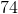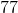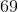Explanation:

Each of the numbers in the leftmost column, or "stems" represents the tens digits of one or more scores; each digit in one of the rows, or "leaf", represents the units digit of a score.

The median score is the score that occurs in the middle when the scores are arranged from least to greatest, which there are, so we count up until we get to the middle score. There are 53, so we look for the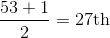highest (or lowest) score. Counting up 27 scores, we get to the "4" in the "7" row, which represents a score of 74. This is the median.

### Example Question #3 : Representing Data

List the data values for the given Stem and Leaf plot.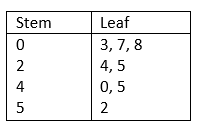3, 7, 8, 24, 25, 45, 52

24, 25, 40, 45, 52

3, 7, 8, 24, 25, 40, 45, 52

2, 5, 25, 30, 42, 52, 70, 80

3, 7, 8, 24, 25, 40, 45, 52

Explanation:

The tens-digits are in the Stem column (left-side) while the ones-digits are in the Leaf column (right-side). Therefore, the data in the Stem and Leaf plot can be written as: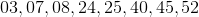### Example Question #4 : Representing Data

List the data values for the given Stem and Leaf plot.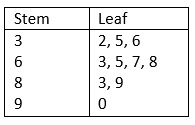32, 35, 36, 63, 65, 67, 68, 83, 89, 90

23, 53, 63, 36, 56, 76, 86, 38, 98, 9

32, 35, 36, 63, 67, 68, 83, 89, 90

32, 35, 36, 63, 65, 667, 68, 83, 89

32, 35, 36, 63, 65, 67, 68, 83, 89, 90

Explanation:

The tens-digits are placed in the Stem column (left-side) and the ones-digits are placed in the Leaf column. Therefore, the data in the Stem and Leaf plot can be written as:### Example Question #5 : Representing Data

List the data values for the given Stem and Leaf plot.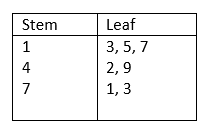13, 15, 17, 42, 49, 71, 73

13, 15, 17, 42, 71, 73

13, 15, 42, 49, 71, 73

31, 51, 71, 24, 94, 17, 37

13, 15, 17, 42, 49, 71, 73

Explanation:

The tens-digits are placed in the Stem column (left-side) and the ones-digits are placed in the Leaf column. Therefore, the data in the Stem and Leaf plot can be written as: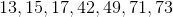### Example Question #6 : Representing Data

List the data values for the given Stem and Leaf plot.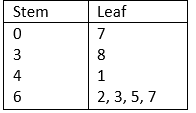07, 38, 62, 63, 65, 67

38, 41, 62, 63, 65, 67

70, 83, 14, 26, 23, 25, 27

7, 38, 41, 62, 63, 65, 67

7, 38, 41, 62, 63, 65, 67

Explanation:

The tens-digits are placed in the Stem column (left-side) and the ones-digits are placed in the Leaf column. Therefore, the data in the Stem and Leaf plot can be written as: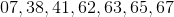### Example Question #7 : Representing Data

Create the Stem and Leaf plot for the given data values.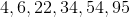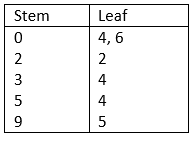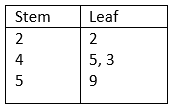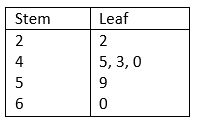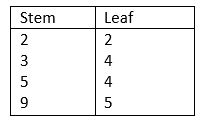Explanation:

The tens-digits are placed in the Stem column (left-side) and the ones-digits are placed in the Leaf column.

The list of values is:

34, 54, 6, 95, 22, 4

This data can also be written as 04, 06, 22, 34, 54, 95### Example Question #8 : Representing Data

Create the Stem and Leaf plot for the given data values:

6, 8, 10, 23, 35, 43, 44, 45, 48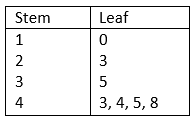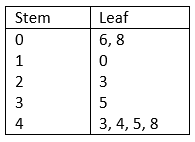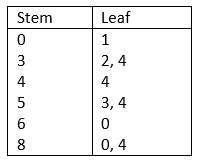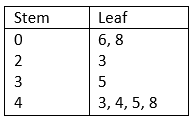Explanation:

The tens-digits are placed in the Stem column (left-side) and the ones-digits are placed in the Leaf column.

The data values are:

6, 8, 10, 23, 35, 43, 44, 45, 48

We can rewrite it as follow

06, 08, 10, 23, 35, 43, 44, 45, 48### Example Question #9 : Representing DataWhich of the following represents the correct median value of the above Stem and Leaf Plot?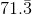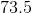Impossible to determine based on the data provided.Explanation:

There are 18 data points in this set, so the median lies halfway between the 9th and 10th sequential data point:

9th Point: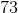10th Point:And: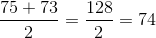The correct median value of the data set is 74

### All Algebra II Resources